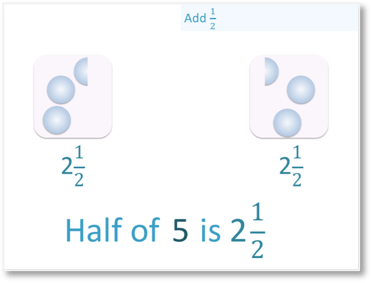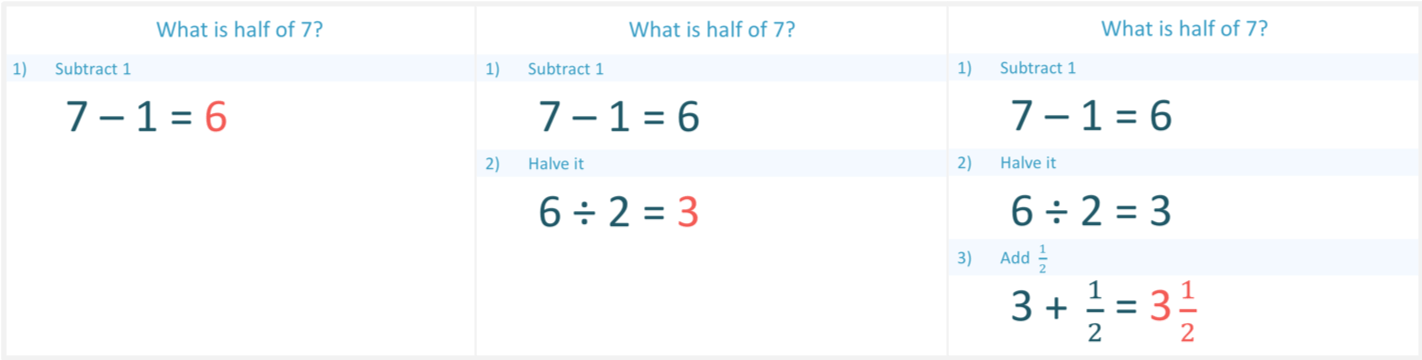# Halving Odd Numbers

Halving Odd Numbers• Odd numbers are not in the two times table and will not make a whole number when we halve them.
• We separate our odd number into the previous even number, plus one.
• 5 = 4 + 1
• We halve the even number, 4, to make 2.
• We halve the remaining one, to make   1 / 2.
• Half of 5 is 2 and a half.
To halve an odd number: subtract one, halve it and then add a half.• 7 is an odd number, which we will halve.
• First we subtract 1 to get an even number.
• 7 – 1 = 6.
• We then find half of 6, which is 3.
• 3 +   1 / 2 = 3   1 / 2.
• Half of 7 is 3 and a half.# Halving Odd Numbers

Odd numbers cannot be halved to give a whole number. We will look at methods we can use for teaching halving odd numbers.

In our first example of halving odd numbers, we are asked to halve the number five. We’ll start by looking at the method of division by sharing. We can represent the number five with five counters. We want to halve it, which means that we want to share it equally across two groups.

We can easily halve four of the counters by placing two in each group, since 4 is even. However, there is one counter remaining in the middle. If we put this whole counter in either of the groups, we would not have shared five equally.

The only way that we can share this last counter equally is to split it in half.

Each half can then be placed in each group. Each group contains 2    1 / 2   counters.

Therefore, half of 5 is 2  1 / 2  .When first introducing halving odd numbers and teaching halving to your child, you could show this easily with plasticine or food, tearing the last item in half.

Starting again with our five counters, we can develop a method to work out halving an odd number mentally.We found that it was easy to halve the counters when the number was even.

We were able to halve four of the counters easily. So, we can begin by subtracting 1 from our odd number.

Subtracting one from every odd number will always give us an even number.

• Step 1: Subtract 15 – 1 = 4

Now that we have an even number, we can easily halve it.

• Step 2: Halve it4 ÷ 2 = 2

We have easily divided the four counters into two groups.

Now, we must remember that one counter that we previously subtracted.

• Step 3: Add  1 / 2The ‘1’ that we originally subtracted must also be halved. Half of 1 is    1 / 2.

So, we must add this on to the 2.

2 +  1 / 2 = 2  1 / 2

Half of 5 is 2  1 / 2.

Another way of thinking of this is that we were able to partition the 5 into 4 + 1.

Half of 4 is 2

Half of 1 is    1 / 2

2 +  1 / 2 = 2 1 / 2

Now we’ll look at another example of halving odd numbers where we will use our three steps.

We are asked to find half of 7.

We can work out this example of halving an odd number by partitioning 7 into 6 + 1.

We do this in the following three steps.• Step 1: Subtract 1

7 – 1 = 6

We subtracted 1 to give us an even number to halve.

• Step 2: Halve it

6 ÷ 2 = 3

• Step 3: Add    1 / 2

3 +    1 / 2 = 3   1 / 2

We add  1 / 2   because this is half of the ‘1’ that we originally subtracted.

We worked this out by partitioning the 7 into 6 + 1. This partitioning method is the easiest to do mentally when teaching your child to halve odd numbers.

First, we halved the 6.

Half of 6 is 3

Next, we halved the 1.

Half of 1 is    1 / 2